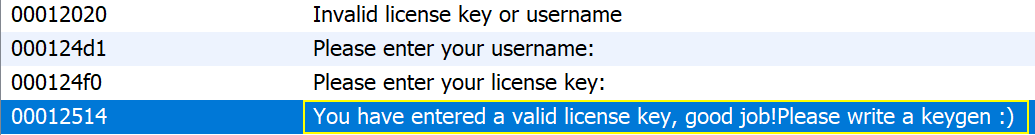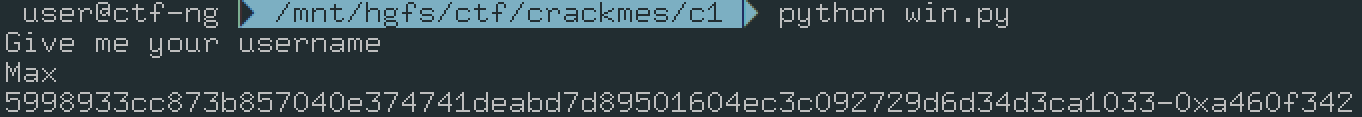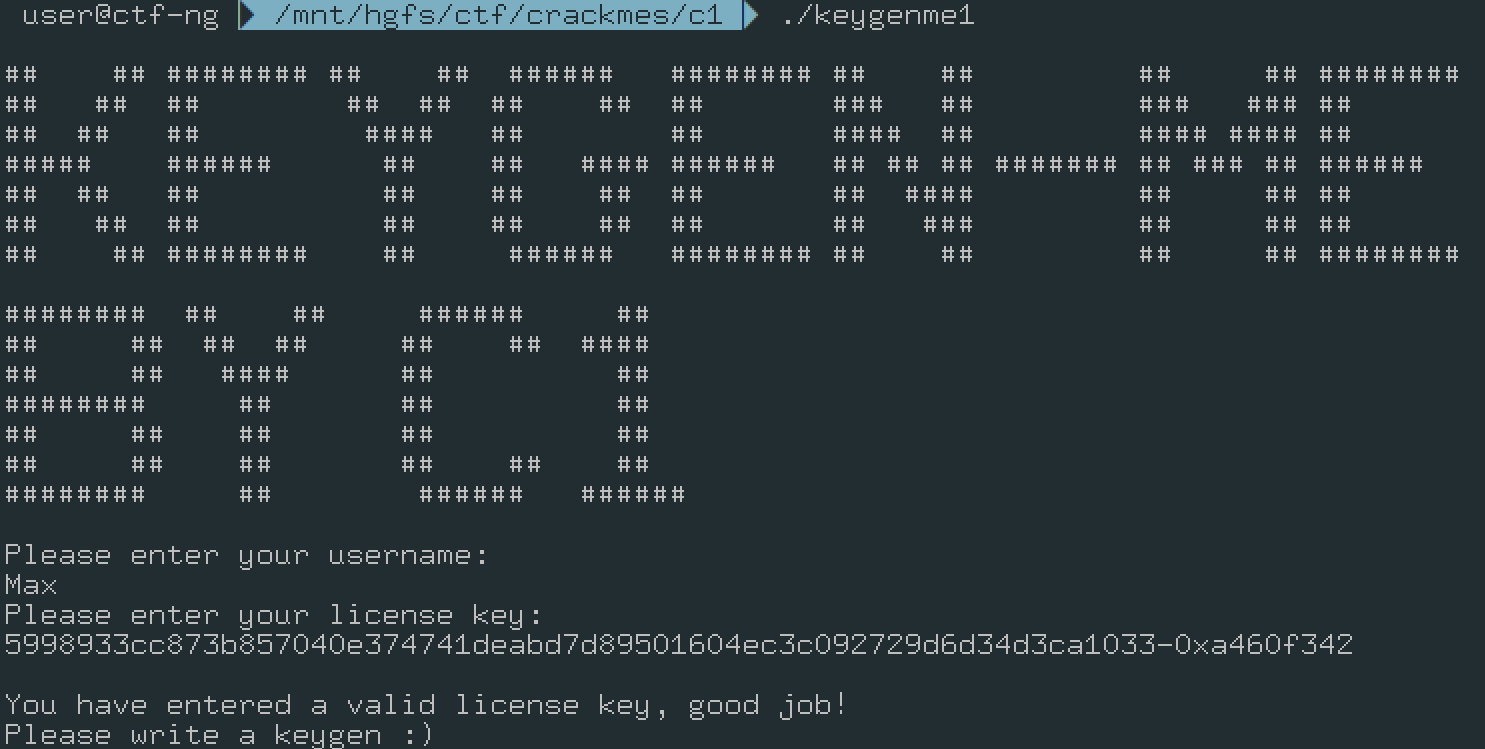# solving c1 keygenme

### 2019-07-04 | Write-up | @maxdebruijn

Keygen information:

 author c1 language c/c++ level 2 platform unix/linux etc. description a easy kegenme, patching not allowed. Have fun!

## Recon

Running the application shows the banner of the crackme and asks the user to input a username and license key. To create a keygen for this application we have to understand the algorithm that is implemented to verify the username and license key. Let’s take a look at the keygenme with Ghidra in order to understand what’s happening with the user input.By looking at the strings in the file 4 strings are present that relate to the keygenme in the defined strings window.The string that is printed when successfully inputting a username and license key is used in the function at `0x000113ef`. To easily locate the string usage select it and use the shortcut `ctrl+shift+f` to find references. The decompilation of this function is as follows.

``````void FUN_000113ef(void)

{
int iVar1;
byte local_148 ;
undefined4 local_128;
char local_124 ;
char local_a4 ;
int local_24;
size_t local_20;
size_t local_1c;
int local_18;
uint local_14;
uint local_10;

local_14 = 0;
local_18 = 0;
memset(local_a4,0,0x80);
memset(local_124,0,0x80);
fgets(local_124,0x7f,stdin);
FUN_00011280(local_124);
fgets(local_a4,0x7f,stdin);
FUN_00011280(local_a4);
local_18 = FUN_00011371(local_a4);
if (local_18 == 0) {
FUN_00011343();
}
local_1c = strlen(local_a4);
local_20 = strlen(local_124);
if (((local_1c == 0x40) && (0 < (int)local_20)) && (iVar1 = FUN_000112b8(local_a4), iVar1 != 0)) {
local_10 = 0;
while (local_10 < 0x20) {
__isoc99_sscanf(local_a4 + local_10 * 2,&DAT_0001250f,&local_128);
local_148[local_10] = (byte)local_128;
local_10 = local_10 + 1;
}
local_14 = FUN_00011219(local_124,local_20);
local_10 = 0;
while (local_10 < 0x20) {
local_24 = 0x1f - local_10;
if (((local_14 >> ((byte)local_24 & 0x1f) ^ (int)(char)local_148[local_10]) & 1) != 0) {
FUN_00011343();
}
local_148[local_10] = (byte)(local_14 >> ((byte)local_24 & 0x1f)) & 1 ^ local_148[local_10];
local_10 = local_10 + 1;
}
iVar1 = FUN_00011219(local_148,0x20);
if (local_18 != iVar1) {
FUN_00011343();
}
puts("\nYou have entered a valid license key, good job!\nPlease write a keygen :)");
return;
}
FUN_00011343();
return;
}
``````

## Cleanup

To continue the analysis it’s usefull to start renaming functions and variables such that the code becomes more readable. The snippet shows that the function at `0x00011343` is often called. By pivoting (simply doubleclick the function) to this function we can see that it’s only purpose is to print `Invalid license key or username` and exit the program. Renaming this function makes it easier to spot which code paths should be avoided, to speed up renaming make use of the shortcut `l` when the variable or function is selected. Other low hanging fruit is renaming the variables where user input is stored to see where it’s used, I do the same for variables that store the output of functions such as `strlen`.

``````void keygenme(void)

{
int iVar1;
int iVar2;
uint uVar3;
byte bVar4;
byte local_148 ;
undefined4 local_128;
uint local_10;

if (iVar1 == 0) {
invalidKey();
}
(iVar2 = FUN_000112b8(license), iVar2 != 0)) {
local_10 = 0;
while (local_10 < 0x20) {
local_148[local_10] = (byte)local_128;
local_10 = local_10 + 1;
}
local_10 = 0;
while (local_10 < 0x20) {
bVar4 = 0x1f - (char)local_10;
if (((uVar3 >> (bVar4 & 0x1f) ^ (int)(char)local_148[local_10]) & 1) != 0) {
invalidKey();
}
local_148[local_10] = (byte)(uVar3 >> (bVar4 & 0x1f)) & 1 ^ local_148[local_10];
local_10 = local_10 + 1;
}
iVar2 = FUN_00011219(local_148,0x20);
if (iVar1 != iVar2) {
invalidKey();
}
puts("\nYou have entered a valid license key, good job!\nPlease write a keygen :)");
return;
}
invalidKey();
return;
}
``````

## RE keysplit

The code shows the first part where the user input is checked and might result in an invalid key message.

``````  iVar1 = FUN_00011371(license);
if (iVar1 == 0) {
invalidKey();
}
``````

Let’s inspect the function at `0x00011371` to see what result it produces and how we can manipulate our input in order for the result to be other than 0.

`````` ulong FUN_00011371(char *param_1)

{
char *pcVar1;
ulong uVar2;

pcVar1 = strrchr(param_1,0x2d); //Gets the last occurence of '-' in the string
if (pcVar1 == (char *)0x0) {
uVar2 = 0; //Return 0 if '-' is not in the string
}
else {
*pcVar1 = 0; //Null out the character '-'
uVar2 = strtoul(pcVar1 + 1,(char **)0x0,0x10); //Take the hex string to the right of '-' and convert it to long
}
return uVar2;
}
``````

The function seems to split the provided key in two parts where the key is divided by the `-` character. After the split the value to the right of `-` is converted to a long and returned by the function. Thus the key will look something like `deadbeef-cafebabe`. A reasonalble name for this function would be getKeyPart2 and the resulting variable keyPart2.

``````void keygenme(void)

{
int keyPart2;
int iVar1;
uint uVar2;
byte bVar3;
byte local_148 ;
undefined4 local_128;
uint local_10;

if (keyPart2 == 0) {
invalidKey();
}
(iVar1 = FUN_000112b8(license), iVar1 != 0)) {
local_10 = 0;
while (local_10 < 0x20) {
local_148[local_10] = (byte)local_128;
local_10 = local_10 + 1;
}
local_10 = 0;
while (local_10 < 0x20) {
bVar3 = 0x1f - (char)local_10;
if (((uVar2 >> (bVar3 & 0x1f) ^ (int)(char)local_148[local_10]) & 1) != 0) {
invalidKey();
}
local_148[local_10] = (byte)(uVar2 >> (bVar3 & 0x1f)) & 1 ^ local_148[local_10];
local_10 = local_10 + 1;
}
iVar1 = FUN_00011219(local_148,0x20);
if (keyPart2 != iVar1) {
invalidKey();
}
puts("\nYou have entered a valid license key, good job!\nPlease write a keygen :)");
return;
}
invalidKey();
return;
}
``````

## RE verify alphabet

The next check asserts that the license length is `0x40` (note that the getKeyPart2 function substituted the `-` for a null byte thus terminating the cstring earlier) and the username must be character or longer. Next to this the license is used by the function at `0x000112b8` and must result in a value other than 0. After cleanup this function looks like the following.

``````undefined4 checkHexNumeric(char *inputString)

{
size_t inputStringLength;
undefined4 returnValue;
ushort **ppuVar1;
size_t inputStringLength2;
uint i;

inputStringLength = strlen(inputString);
if ((inputStringLength & 1) == 0) {
i = 0;
while (inputStringLength2 = strlen(inputString), i < inputStringLength2) {
ppuVar1 = __ctype_b_loc();
if (((*ppuVar1)[(int)inputString[i]] & 0x1000) == 0) {
return 0;
}
i = i + 1;
}
returnValue = 1;
}
else {
returnValue = 0;
}
return returnValue;
}
``````

The function iterates over all character in the provided string and checks it for a specific trait. The `__ctype_b_loc` function results in a onehot encoded value showing if a byte is printable, numeric, uppercase, etc. where in this case it’s checked if the byte represents a hex numeric value. This confirms that the first part of the license has to be hexadecimal as well.

## RE binary transformation and crc checksum

Directly after this assertion we see the following code snippet

``````i = 0;
while (i < 0x20) {
local_148[i] = (byte)local_128;
i = i + 1;
}
``````

The license key is transformed from hex to binary with the use of sscanf so let’s name the variable accordingly.

After the transformation the username and username length are provided to the function at `0x00011219` so let’s find out what it does.

``````uint FUN_00011219(int param_1,uint param_2)

{
uint i;
uint local_c;

local_c = 0xffffffff;
i = 0;
while (i < param_2) {
local_c = *(uint *)(&DAT_00012560 + (((uint)*(byte *)(i + param_1) ^ local_c) & 0xff) * 4) ^
local_c >> 8;
i = i + 1;
}
return ~local_c;
}
``````

This snippets implements a crc32 calculation.

``````uint crc32(int inputString,uint inputStringLength)

{
uint i;
uint crc32;

crc32 = 0xffffffff;
i = 0;
while (i < inputStringLength) {
crc32 = *(uint *)(&DAT_00012560 + (((uint)*(byte *)(i + inputString) ^ crc32) & 0xff) * 4) ^
crc32 >> 8;
i = i + 1;
}
return ~crc32;
}
``````

## RE input validation algorithm

``````i = 0;
while (i < 0x20) {
bVar2 = 0x1f - (char)i;
if (((usernameCrc32 >> (bVar2 & 0x1f) ^ (int)(char)binaryLicense[i]) & 1) != 0) {
invalidKey();
}
i = i + 1;
}
``````

Each byte provided by the license key is checked. The check verifies that the bit in the crc32 of the username corresponding the the byte in the license has the same last bit. Afterwards a variation of this calculation is stored in place.

Finally the crc32 of the binary key is calculated and compared to KeyPart2.

``````void keygenme(void)

{
int keyPart2;
int iVar1;
byte bVar2;
undefined4 local_128;
uint i;

if (keyPart2 == 0) {
invalidKey();
}
(iVar1 = checkHexNumeric(license), iVar1 != 0)) {
i = 0;
while (i < 0x20) {
i = i + 1;
}
i = 0;
while (i < 0x20) {
bVar2 = 0x1f - (char)i;
if (((usernameCrc32 >> (bVar2 & 0x1f) ^ (int)(char)binaryLicense[i]) & 1) != 0) {
invalidKey();
}
i = i + 1;
}
invalidKey();
}
puts("\nYou have entered a valid license key, good job!\nPlease write a keygen :)");
return;
}
invalidKey();
return;
}
``````

## Writing the keygen

Now that we have a proper understanding of the algorithm used to check the user input we can finally start keygenning. The goal is to build a valid key based on the username provided to the keygen. We know that the license is dependent on the username and the second part of the key is a crc checksum over the binaryLicense. Thus we have to take the following steps to build the keygen.

1. Get the username and calculate the crc checksum
2. Build the first part of the key based on the checks against the username checksum.
3. Calculate the checksum of the binarKey
4. Provide the user with the full license details.

For most of these steps there are default libraries available that will help building the keygen, the only challenge is manipulating the first part of the key. The following pseudocode implements the check on the first part of the key.

``````bVar2 = 0x1f - i #Where i is the location of the byte in the key
verification = user_crc32 >> (0x1f & bVar2)
if (keypart ^ verification) & 1 == 0:
print("The chosen byte satisfies the algorithm")
``````

If our chosen byte doesn’t satisfy the algorithm we can simply flip the last bit to do so. Using this we get the following keygen.

``````import binascii
from random import randint

key = bytearray()
calculatekey = bytearray()
for i in range(0x20):
keypart = randint(0,255)
bVar2 = 0x1f - i
verification = user_crc32 >> (0x1f & bVar2)
if (keypart ^ verification) & 1 == 0:
key.append(keypart)
calculatekey.append((user_crc32 >> (0x1f & bVar2))&1^keypart)
else:
key.append(keypart^1)
calculatekey.append((user_crc32 >> (0x1f & bVar2))&1^keypart^1)

keypart1 = binascii.hexlify(key).decode()
keypart2 = hex(binascii.crc32(calculatekey))
print("{}-{}".format(keypart1, keypart2))
``````

Running this script gives the following output for example.Using the output from the keygen we get the following output from the keygemne.